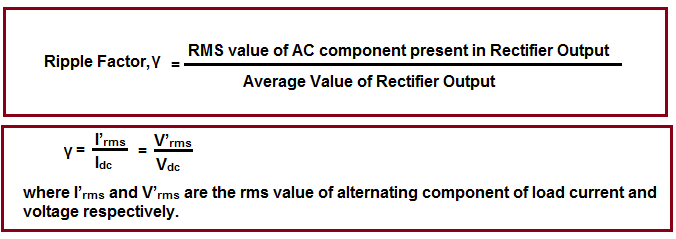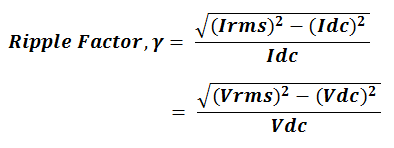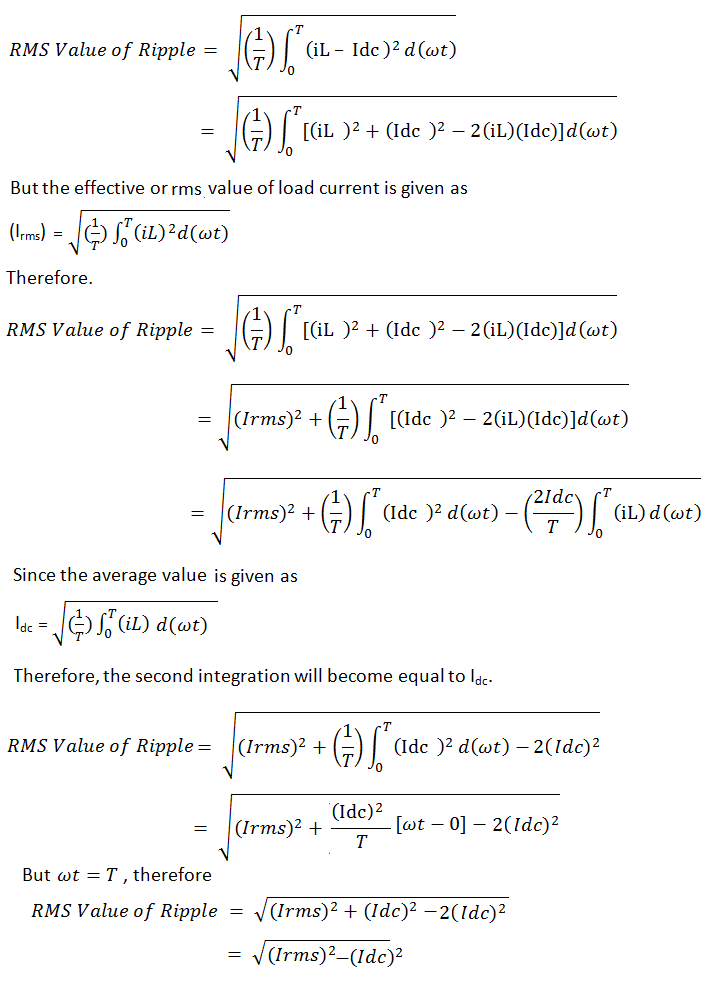# What is Ripple and Ripple Factor? – Formula of Ripple Factor

### What is Ripple?

Ripple is the fluctuating AC component present in rectified DC output. The output of a rectifier may either be DC current or voltage. In view of this, AC fluctuating component present in DC output voltage is called voltage ripple and that in DC current output is called current ripple.

#### Why Ripple is Present?

Ripple is always present in the rectifier output. This is because of the behavior of circuit elements like diode or thyristor. Let us consider an example of single phase full wave rectifier to better understand the reason for presence of ripple. The output current waveform of single phase full wave rectifier is shown below.The above figure is self-explanatory. The expected output from full wave rectifier should be a pure DC but actually the output current is different. Since the average value of output current is the pure, therefore the ripple present is equal to the difference of output current or load current minus average current.

### What is Ripple Factor?

Ripple Factor is the ratio of rms value of ac component present in the rectified output to the average value of rectified output. It is a dimensionless quantity and denoted by γ. Its value is always less than unity.This is basically a measurement of ripple which denotes the purity of rectified output. The more the ripple factor, the lesser will be the purity of rectifier DC output. This means more will be fluctuating AC component. Thus every effort is made to reduce the ripple factor. Here we will not discuss the ways to reduce the ripple factor.

Ripple factor is generally denoted in percentage like 3 % or 4 %. Percentage ripple factor is obtained by just multiplying γ by 100. 3 % ripple content in output current means that 3 A rms alternating component of current is present against the actual 100 A DC current output. Similarly, 3 % ripple content in output voltage means that 3 V rms alternating component of voltage is present against the actual 100 V DC voltage output.

### Ripple Factor Formula

The formula for ripple factor is given in terms of rms value and average value of rectifier output.#### Derivation of Ripple Factor Formula

The ripple factor formula can easily be derived from its definition. As per definition, we need to find two parameters: rms value of ripple present in rectifier output current or voltage and average value of output of rectifier for one time period T. For calculating rms value of ripple Irms, first we need to find the ripple. Ripple is equal to the difference between the actual output minus expected DC output. Thus,

Ripple = iL – Idc = vL – Vdc

Here, iL and vL are the output current and voltage through the load connected at the terminals of rectifier. Similarly, Vdc and Idc are the average value of load voltage and current.

Let us now find the rms value of current ripple. Though rms value of voltage ripple can also be used for deriving the formula for ripple factor but here we are using current ripple.Since the ripple factor is equal to the ratio of rms value of ripple content and average value of rectifier output, therefore its formula becomesHope, ripple and ripple factor is clear. If you have any doubt, please write in comment box.

### 11 thoughts on “What is Ripple and Ripple Factor? – Formula of Ripple Factor”

1.Vijaya says:

I dc value will not contain square root but in your derivation part you have shown that.
(2idc) whole square is shown but here only idc
Should be squared

1.admin says:

Thank you very much for your correcting the typo. Please check, it has been corrected.

2.Aram says:

Hello:

I am trying to rule out power supply noise bleeding into my circuitry, so as a side task, I want to verify how good my power supplies are. I found this link but I would like someone to verify I did the measurements and calcs correctly:

Step 1- Used a 5.5 digit DMM, and measured the DC ouput. For this example, it measured: 12.11 VDC.
Step 2- Used a 5.5 digit DMM and verified with a scope the the A/C component which measured: 230uV rms. Quite low me thinks.

I calculated the ripple factor (not percentage):

(See last ripple equation you provided above)

So in the article it says to convert the factor to %, simply multiply by 100. Okay I did…
So now: 99.999999918 %

So that is telling me my ripple is 99.99………%? I don’t think so, it most likely tells me that the percentage is that much in DC.

Now I have to admit in my calculations, on the top line of the equation, the number comes out negative, so I square rooted the absolute value

Where did I go wrong? How do I calculate the actual ripple in percent?

Help very much appreciated.

1.admin says:

carefully read the definition of ripple factor. It says, it is the ratio of rms value to the average value. Here rms value is the root mean suare value of rectifier output which is having DC and AC mixed. You used DMM in AC mode and measured 230uV, so this reading is not the rms value of the output rather it is rms value of ac component present in the output. Your ripple calculation should be,
Ripple factor = (230uV/12.11)x100 = 0.0019%.

Please comment if you have any thing to share. Pleas share if you like it.

3.barry says:

please can you simplify it so it can be easier to understand

4.Cadris Silver says:

What is the real physical implementation(meaning) of the term “Ripple Factor”?

1.admin says:

When you have DC, you are sulposed to have 100% DC. Bud generator do not produce DC rather AC is converted in DC by using commutator or rectifier. So we need to know, how much ac component is there in the DC. This is where ripple factor come into picture.

5.Shashwat Tripathi says:

Very well explained
Thanks

6.Yamini says:

Thankyou so much, you explained it very nicely.

7.atom faraday says:

nice article

8.SHREE ELECTRONICS, pramod V Patil says:

For example
Output DC voltage is 250.0 vdc
Output current is 100.0 amp dc
Then give me formula to calculate
Ripple factor, ripple percentage
Ripple Content in output DC voltage

This site uses Akismet to reduce spam. Learn how your comment data is processed.